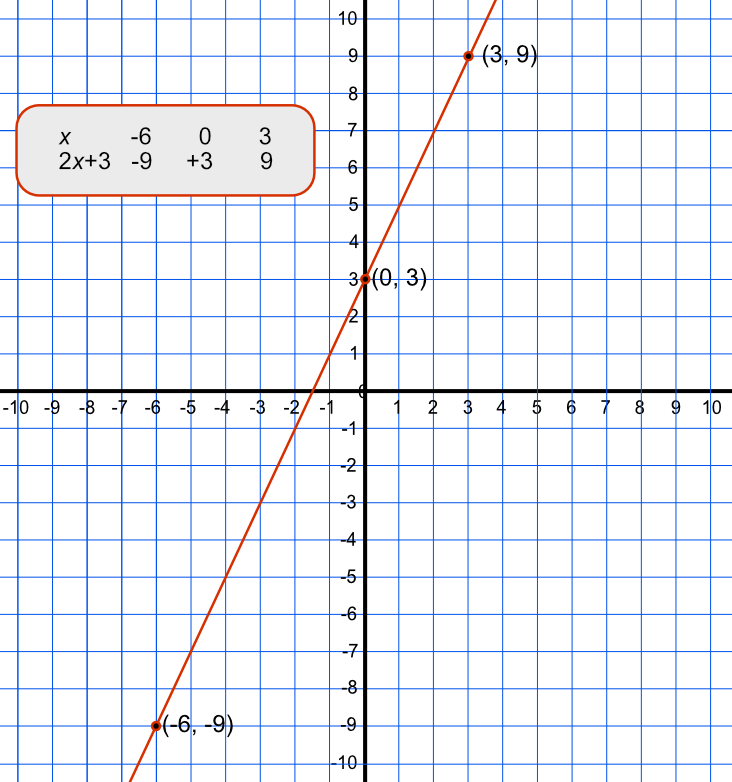Plotting Graphs of Straight Lines

# Plotting Graphs of Straight Lines

GCSE(F),

To plot a straight line, create a small table.

Determine at least three values of x to plot, and work out the values of y using the equation. Plot the points, and draw a straight line through the three points, extending the graph beyond the two outside points.

If a straight line cannot be drawn through these points, check the calculations.

## Examples

1. An equation is given by y = 3x - 4.

What are the coordinates that would be plotted for x = -2, x = 0 and x = 3?

Answer: (-2, -10), (0, -4) and (3, 5)

 x -2 0 3 3x - 4 -10 -4 5

2. Plot the graph for y = 2x + 4.

Answer: